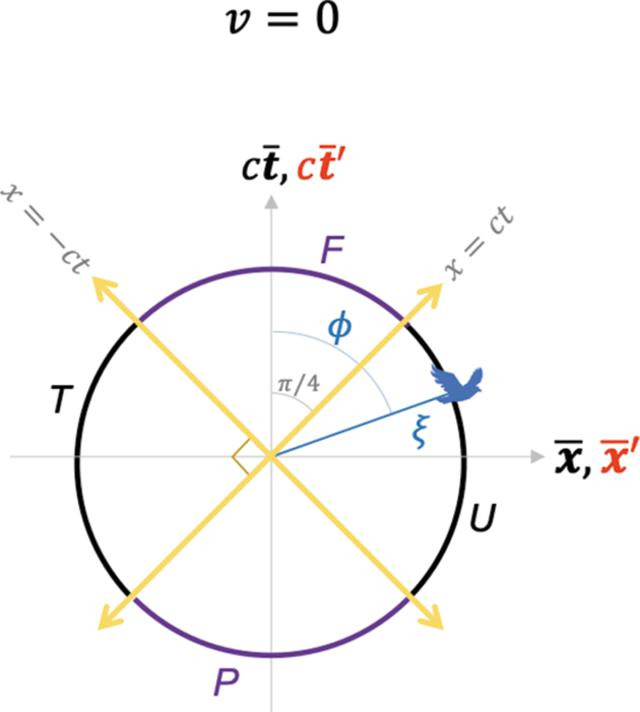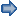disable zoom     view article Figure 6 A special case of the blended and the RBS coordinates, equation (11b), where v = 0. RBS coordinates, given in equation (11b), transform the blended coordinates plot in Fig. 4into a circle of radius, where the four hyperbola branches in Fig. 4become four arc segments of the circle here. Purple (black) arc segments represent time-like (space-like) events. Light lines are shown by yellow lines. See the Mathematica script in the Mathematica notebook in the supporting information to generate this plot.FOUNDATIONSADVANCES
ISSN: 2053-2733
Volume 77| Part 4| July 2021| Pages 242-256